UPSC  >  Test: Logical Sequence- 1

# Test: Logical Sequence- 1 - UPSC

Test Description

## 10 Questions MCQ Test CSAT Preparation - Test: Logical Sequence- 1

Test: Logical Sequence- 1 for UPSC 2023 is part of CSAT Preparation preparation. The Test: Logical Sequence- 1 questions and answers have been prepared according to the UPSC exam syllabus.The Test: Logical Sequence- 1 MCQs are made for UPSC 2023 Exam. Find important definitions, questions, notes, meanings, examples, exercises, MCQs and online tests for Test: Logical Sequence- 1 below.
Solutions of Test: Logical Sequence- 1 questions in English are available as part of our CSAT Preparation for UPSC & Test: Logical Sequence- 1 solutions in Hindi for CSAT Preparation course. Download more important topics, notes, lectures and mock test series for UPSC Exam by signing up for free. Attempt Test: Logical Sequence- 1 | 10 questions in 10 minutes | Mock test for UPSC preparation | Free important questions MCQ to study CSAT Preparation for UPSC Exam | Download free PDF with solutions
 1 Crore+ students have signed up on EduRev. Have you?
Test: Logical Sequence- 1 - Question 1

### I wear a pad every time I bat. (i) I batted. (ii) I didn’t bat. (iii) I wore a pad. (iv) I didn’t wear a pad.

Detailed Solution for Test: Logical Sequence- 1 - Question 1

If I wear a pad every time I bat, then it also shows that if I did not wear a pad, I did not bat. So, (ii) follows (iv) logically.
Please note that wearing a pad does not mean I batted. Logic is given other way round, that If I bat, then I wear a pad.
I may wear a pad, but do not bat. Correct option is (d)

Test: Logical Sequence- 1 - Question 2

### A poem has seven lines, each of which starts with the letters P, Q, R, S, T, U and V. The line starting with R is the third from the bottom and the line starting with V is the second from the top. The line starting with Q and that starting with U have exactly three lines in between. Either S or P is the only letter between R and U. The poem is likely to start with

Detailed Solution for Test: Logical Sequence- 1 - Question 2

It is given that R is 3rd from the bottom and V is 2nd from the top, then R and U have only one line between them, i.e. either S or P. Now the possible arrangement will be as Follows.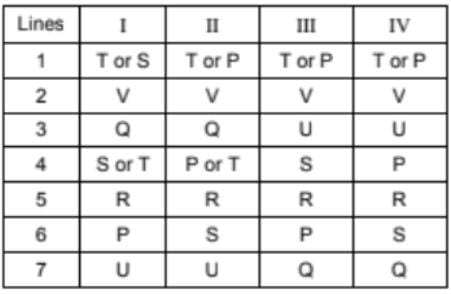So, T or S or P has to be in line 1 and the poem starts with T or S or P.

Test: Logical Sequence- 1 - Question 3

### K, L, M, N, O and P are six trees in a garden. Neither M nor N is the tallest tree. Tree L is taller than P but shorter than tree K. Tree O is as tall as P but taller than M. Which of the following trees is the tallest?

Detailed Solution for Test: Logical Sequence- 1 - Question 3

Neither tree M nor tree N is the tallest.
Tree L is taller than tree P but is shorter than tree K,
whereas tree O is as tall as tree P, but taller than tree M.
So, the order may be as follows
1    2    3   4
K    L   P   M
-     -    O   -
As the tree N is not the tallest, K must be the tallest. Choice (b)

Test: Logical Sequence- 1 - Question 4

I will buy the airplane only if it is the costliest and the fastest.

Detailed Solution for Test: Logical Sequence- 1 - Question 4

If I bought aeroplane, it would be the fastest & the costliest. If I did not buy the aeroplane it is either not the fastest or not the costliest or neither fastest nor the costliest (not necessarily neither fastest nor the costliest). Only option (c) show a feasible case of buying the aeroplane

Test: Logical Sequence- 1 - Question 5

Six persons A, B, C, D, E and F leave for the station in such a way that C leaves before F leaves and after D leaves, whereas E leaves before B leaves, who leaves before F leaves, but A leaves after both F and C leave. Who is the last person to leave?

Detailed Solution for Test: Logical Sequence- 1 - Question 5

As it is given that C leaves the station before F leaves, but after D leaves, i.e. D > C > F.
So D C F can be the order of their departure.
E leaves the station before B leaves, who leaves before
F leaves, i.e. E > B > F.
So E B F can be the order of their departure.
A leaves the station after F and C leave the station,
i.e. C > F> A.
So A is the last person to leave as shown below.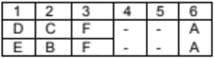Test: Logical Sequence- 1 - Question 6

If the milk is not cold then I will not go to school and will not have dinner.

Detailed Solution for Test: Logical Sequence- 1 - Question 6

If the milk is not cold then I will not go to the school & will not have dinner. Then, if I had gone to school or I had dinner, the milk is cold. Correct option is (c)

Test: Logical Sequence- 1 - Question 7

These questions are based on the following information.
P, Q, R are three girls and A, B, C are three boys.
Q is taller than R but shorter than C, who is taller than A.
P is taller than B, who is taller than C.

Who is the tallest of all the six?

Detailed Solution for Test: Logical Sequence- 1 - Question 7

The given conditions are as follows.
P, Q, R are three girls.
A, B, C are three boys.
Q is taller than R i.e. Q > R.
C is taller than Q i.e. C > Q.
C is taller than A i.e. C > A.
P is taller than B i.e. P > B.
B is taller than C i.e. B > C.
Now, the arrangement can be as follows.
C > Q > R and P > B > C and C > R.
Now, after considering all the above given conditions, the
The arrangement is as follows.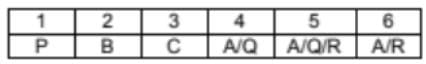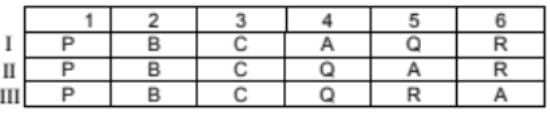P is the tallest of all.

Test: Logical Sequence- 1 - Question 8

These questions are based on the following information.
P, Q, R are three girls and A, B, C are three boys.
Q is taller than R but shorter than C, who is taller than A.
P is taller than B, who is taller than C.

Who is the shortest of the three girls?

Detailed Solution for Test: Logical Sequence- 1 - Question 8

The given conditions are as follows.
P, Q, R are three girls.
A, B, C are three boys.
Q is taller than R i.e. Q > R.
C is taller than Q i.e. C > Q.
C is taller than A i.e. C > A.
P is taller than B i.e. P > B.
B is taller than C i.e. B > C.
Now, the arrangement can be as follows.
C > Q > R and P > B > C and C > R.
Now, after considering all the above given conditions, the
The arrangement is as follows.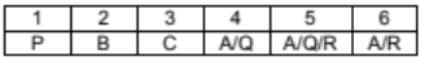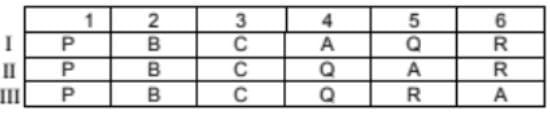R is the shortest among the three girls. P > Q > R.

Test: Logical Sequence- 1 - Question 9

You cannot clear the CAT unless you are hard working.
(i) You are hard working.
(ii) You can clear the CAT.
(iii) You are not hard working.
(iv) You cannot clear the CAT.

Detailed Solution for Test: Logical Sequence- 1 - Question 9

If you cannot clear the CAT unless you are hardworking, then if you are not hardworking you cannot clear the CAT. So, (iv) follows (iii) logically Correct option is (d)

Test: Logical Sequence- 1 - Question 10

Whenever my mother scolds me, I either hide behind my father or complain to my grandma.

Detailed Solution for Test: Logical Sequence- 1 - Question 10

If whenever my mother scolds me, I either hide behind my father or complain to my grandma. Then, if I did not complain to my grandma & did not hide behind my father, my mother must not have scolded me. Correct option is (a)

## CSAT Preparation

197 videos|151 docs|200 tests
Information about Test: Logical Sequence- 1 Page
In this test you can find the Exam questions for Test: Logical Sequence- 1 solved & explained in the simplest way possible. Besides giving Questions and answers for Test: Logical Sequence- 1, EduRev gives you an ample number of Online tests for practice

## CSAT Preparation

197 videos|151 docs|200 tests

### How to Prepare for UPSC

Read our guide to prepare for UPSC which is created by Toppers & the best Teachers